### Home > CCA > Chapter 8 > Lesson 8.1.1 > Problem8-9

8-9.

Remember that a Diamond Problem is a pattern for which the product of two numbers is placed on top, while the sum of the same two numbers is placed on bottom. (This pattern is demonstrated in the diamond at right.) Copy and complete each Diamond Problem below.1.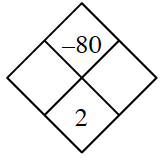What two numbers add to $2$ and multiply to $−80$?

1.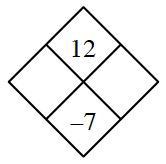For parts (b) through (d) refer to the hint given in part (a).

1.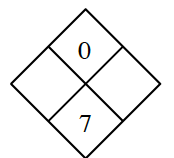1.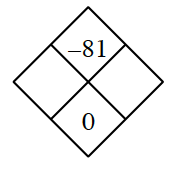1.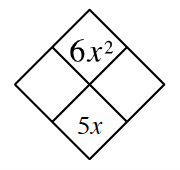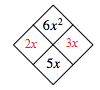1.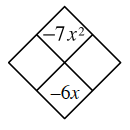Think of two numbers that multiply to $-7$ and add to $-6$.
Also refer to answer in part (c).